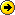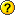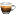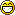Page 2 of 7

### Re: User Functions Exchange

Posted: 24 Apr 2015 10:37
typoini.inc or inc.incCorrected### Re: User Functions Exchange

Posted: 25 Apr 2015 07:07
getlayout(element, separator) : returns value of a UI layout element. Multiple elements can be specified, separated by separator (default is ","). Return is also separated by the same.
Use native function setlayout() for available layout element names.

function

Code: Select all

``````FUNCTION getlayout(\$elems, \$sep='|') {
// Returns value of one or more layout elements.
//   \$elems  one or more layout element names, separated by \$sep
//           valid element names can be found in return of setlayout().
//   \$sep    separator between multiple elements. Default is "|"
// multiple return values are separated by \$sep.

foreach (\$elem, \$elems, \$sep) {
if (\$elem == '') || (regexmatches(\$elem,'^\w[\W]') != '') {
\$ret = (isset(\$ret) ? \$ret : '') . \$sep . '' ; //no value for bad elem
} else {
\$val = gettoken(regexmatches(setlayout(), "\b\$elem\=\d*", 1), 2, '=');
\$ret = (isset(\$ret) ? \$ret : '') . \$sep . \$val;
}
}
return replace(\$ret, \$sep, '',,, 1); //replace first extra \$sep
}``````
call
assuming the function getlayout() is saved in <xyscripts>\inc\inc.xyi

Code: Select all

``````include "inc\inc.xyi";
eval();
text getlayout("ShowMainMenu,InfoPanelHeight"); //returns "1,400" for example
text getlayout("ShowMainMenu|InfoPanelHeight", '|'); //returns "1|400" for example``````

### Re: User Functions Exchange

Posted: 29 Apr 2015 19:53
dict() : a ~dictionary/hash function. Description in function comment.

function

Code: Select all

``````FUNCTION dict(\$var, \$key = '', \$seprec = "<crlf>", \$sepfld = '|', \$isindex = 0, \$case = 0, \$keynum = 1) {
/*Poor man's dictionary for XY, as a function.
* say \$var is: "Key1|Data1<crlf>Key2|Data2"
* then dict(\$var,'Key1') will return "Data1"
*   \$var      the array container variable
*   \$key      the key name.
*   \$seprec   separator between (key, data) records. Default <crlf>
*   \$sepfld   separator between key and data fields. Default |
*   \$isindex  \$key is a (1-based) index. Find the key in this position. 0=no, 1=yes. Default 0
*   \$case     lettercase sensitivity for \$key, \$seprec, \$sepfld. 1=yes, 0=no. Default 0
*   \$keynum   if multiple keys exist of same \$key name, find this one among them
* \$key can be empty and will try to match empty key.
* if \$keynum is defined as > 1,but that many matched keys do not exist, '' is returned
* the data cannot have \$seprec, but can have \$sepfld. \$key can have neither. */

//work-around for an inconvenience in processing default values of UDF params
//not needed since v15.00.0518
//\$var = \$var, 'r'; \$key = \$key, 'r'; \$seprec = \$seprec, 'r'; \$sepfld = \$sepfld, 'r';
\$return = '';
if (\$isindex == 1) {
\$rec    = gettoken(\$var, \$key, \$seprec);
if (\$rec != '') {\$return = gettoken(\$rec, 2, \$sepfld,, 2); }
}
else {
\$keypos    = strpos(\$seprec.\$var, \$seprec.\$key.\$sepfld,, \$case);
if (\$keypos != -1) {
while (\$keynum > 1) {
\$keypos = strpos(\$seprec.\$var, \$seprec.\$key.\$sepfld, \$keypos + strlen(\$seprec.\$key), \$case);
\$keynum--;
}
}
if (\$keypos != -1) {
\$databgn = strlen(\$key.\$sepfld) + \$keypos ;
\$datalen = strpos(\$var.\$seprec, \$seprec, \$keypos,, \$case) - \$databgn ;
if (\$datalen > 0) { \$return  = substr(\$var, \$databgn, \$datalen); }
}
}
return \$return;
}
``````
[/size]

call
assuming dict() is saved in <xyscripts>\inc\inc.xyi :

Code: Select all

``````include 'inc\inc.xyi'
\$table = <<<#lit
key1    data1
key2    data21    data22
key3    data3
key4        data4
34
key3    second key3 data#lit;
text dict(\$table,'key2',,'    '); //default
text dict(\$table,4,,'    ',1); //find key by index
text dict(\$table,'',,'    '); //match empty key
text dict(\$table,'Key1',,'    ',,1); //case-sensitive
text dict(\$table,'key3',,'    ');  //take first matching key
text dict(\$table,'key3',,'    ',,,2); // take second matching key``````
This probably needs some testing...
 hey, if it allowed adding or removing keys, it can almost become an array function!

[update]
added an experimental parameter, \$keynum, to decide which key to process when there are multiple keys of same \$key name.
Experimental because it has a couple catches:
1) if \$keynum points to a non-existent matching-key count, nothing is returned. Make sure there are so many matches before defining \$keynum.
2) If an earlier key doesn't have field-separators (\$sepfld) after it, it's skipped by the counter. (but I guess that can be called a syntax error in \$var)

### Re: User Functions Exchange

Posted: 30 Apr 2015 17:13

Code: Select all

``````function hextobin(\$hex) {
// Returns the binary form of an hexadecimal number.
// Numbers with spaces are accepted, and such spaces
// are NOT removed after the conversion. If any other
// character besides 0-9, a-f (case insensitive) and single
// white spaces is present, the function throws an error
// and aborts.
//
//   \$hex   the hexadecimal number to convert

assert regexmatches("\$hex", "[^0-9a-f ]") == "", "Argument is not a valid hexadecimal number";
return replacelist("\$hex", "0,1,2,3,4,5,6,7,8,9,a,b,c,d,e,f", "0000,0001,0010,0011,0100,0101,0110,0111,1000,1001,1010,1011,1100,1101,1110,1111", ",");
}``````
Question: how can I throw an error in case \$hex isn't a proper hex number?

### Re: User Functions Exchange

Posted: 30 Apr 2015 17:19
Marco wrote:

Code: Select all

``````function hextobin(\$hex) {
// Returns the binary form of an hexadecimal number
//
//   \$hex   the hexadecimal number to convert

return replacelist("\$hex", "0,1,2,3,4,5,6,7,8,9,a,b,c,d,e,f", "0000,0001,0010,0011,0100,0101,0110,0111,1000,1001,1010,1011,1100,1101,1110,1111", ",");
}``````
Question: how can I throw an error in case \$hex isn't a proper hex number?

Code: Select all

``Assert RegexReplace(\$hex, '[A-F0-9]') == '', 'Hex is not valid.';``
Add any other characters you want to accept (like spaces) to the character class.

### Re: User Functions Exchange

Posted: 30 Apr 2015 17:23
Assert! Didn't think of that! Thank you!

### Re: User Functions Exchange

Posted: 01 May 2015 15:38
And here's a cousin of Marco's hextobin.

dectohex() : convert a decimal/base-10 positive integer number to hexadecimal/base-16.
function

Code: Select all

``````FUNCTION dectohex(\$n) {
// convert a decimal positive integer \$n to hex. \$n is rounded and "unsigned' if needed
\$int = abs(round(\$n));  \$rem = 0;  \$return = '';
while (\$int > 0) {
\$r = (\$int/16);
\$int = gettoken(\$r, 1, '.');
\$rem = '.' . gettoken(\$r, 2, '.');
\$h = (\$rem*16);
\$h = replacelist(\$h,'10|11|12|13|14|15', 'A|B|C|D|E|F', '|') ;
\$return = \$h . \$return;
}
return \$return;
}``````
call
assuming dectohex() is saved in <xyscripts>\inc\math.xyi

Code: Select all

``````INCLUDE "inc\math.xyi"
echo dectohex(2046); //returns 7FE
echo dectohex('89') //returns 59``````

ed. this version (posted earlier) requires following two int() and frac() functions in math.xyi and under namespace math

Code: Select all

``````NAMESPACE math
FUNCTION dectohex(\$n) {
\$rem = 0; \$return = '';
while (\$int > 0) {
\$r = (\$int/16);  \$int = math::int(\$r); \$rem = math::frac(\$r, 1);
\$h = (\$rem*16);
\$h = replacelist(\$h,'10|11|12|13|14|15', 'A|B|C|D|E|F', '|') ;
\$return = \$h . \$return;
}
return \$return ;
}``````
[/size]

ed. didn't return for 0.
ed. was broken. also removed lasterr error-capture

### Re: User Functions Exchange

Posted: 01 May 2015 15:43
int(), frac(), sign() : returns resp. integer part, fractional part, or sign of a decimal number.
functions

Code: Select all

``````NAMESPACE math
FUNCTION int(\$n) {
// returns integer part of a decimal number \$n
\$return = gettoken(\$n, 1, '.');
return \$return *1 ;
}

FUNCTION frac(\$n, \$dot = 0) {
// returns fractional part of a decimal number \$n, with decimal point if \$dot == 1
\$return = ((\$dot == 0) ? '' : '.') . gettoken(\$n, 2, '.');
return \$return *1 ;
}

FUNCTION sign(\$n){
// returns the sign of a decimal number as either '-' or '+'.
\$p = substr(\$n, 0, 1);
return (\$p Like "[-+]") ? \$p : '+';
}

``````
call
assuming these are saved in <xyscripts>\inc\math.xyi

Code: Select all

``````INCLUDE inc\math.xyi
"test"
echo frac(5.67, 1); //.67
echo frac(5.67);    //67
echo int(-5.67);    //-5
echo int(.25);      //0
echo sign(5);       //+
echo sign(4-5);     //-``````
ed. didn't return for 0.
ed. included sign()

### Re: User Functions Exchange

Posted: 01 May 2015 17:34
fact() : Factorial . Returns the factorial of an integer. (Only reliable upto about 20! )

nPr() : Permutation. Returns count of unique ways to pick \$r elements, ordered uniquely, from a set of \$n elements.
nCr() : Combination. Returns count of unique ways to pick \$r items out of \$n in any order.
Keep both \$n and \$r at or under the 10's for best reliability. (And approx. < 28 for any reliability) Must be true: \$n > \$r; \$n > 0; \$r > 0

Note that as the functions are posted here, nPr and nCr both require fact() to exist under the same namespace. otherwise intelligently replace math::fact() with the code for fact().
function

Code: Select all

``````NAMESPACE math
FUNCTION fact(\$n) {
//return factorial of \$n. Keep \$n in the < 20's
global \$lasterr = '';
if (\$n*1 == 0) && (\$n != 0 ){ \$lasterr = 'fact::NaN'; return 0; }
if (\$n > 20) { \$lasterr = 'fact::ResultMaybeOff'; } //fair warning
\$n = abs(round(\$n)); \$r = 1; if (\$n == 0) { \$n = 1; }
while (\$n > 0){ \$r = \$r * \$n; \$n--; }
return \$r *1 ;
}

FUNCTION nCr(\$n, \$r) {
//return combination-count of \$r items out of \$n. Keep \$n and \$r in the < 28's. \$n, \$r is silently rounded to +\$n and +\$r
global \$lasterr = '';
if (\$n*1 == 0) && (\$n != 0 ){ \$lasterr = 'nCr::nNaN'; return ''; }
if (\$r*1 == 0) && (\$r != 0 ){ \$lasterr = 'nCr::rNaN'; return ''; }
\$n = abs(round(\$n)); \$r = abs(round(\$r));
if (\$n < \$r)                { \$lasterr = 'nCr::BADINPUT'; return ''; }
if (\$n > 12) || (\$r > 12)   { \$lasterr = 'ncr::ResultMaybeApprox'; } //fair warning
return round((math::fact(\$n) / (math::fact(\$r) * math::fact(\$n - \$r))) *1) ;
}

FUNCTION nPr(\$n = 1, \$r = 1) {
//return permutation-count of \$r items out of \$n. Keep \$n and \$r in the < 28's. \$n, \$r is silently rounded to +\$n and +\$r
global \$lasterr = '';
if (\$n*1 == 0) && (\$n != 0 ){ \$lasterr = 'nPr::nNaN'; return ''; }
if (\$r*1 == 0) && (\$r != 0 ){ \$lasterr = 'nPr::rNaN'; return ''; }
\$n = abs(round(\$n)); \$r = abs(round(\$r));
if (\$n < \$r)                { \$lasterr = 'nPr::BADINPUT'; return ''; }
if (\$n > 20) || (\$r > 20)   { \$lasterr = 'nPr::ResultMaybeOff'; } //fair warning
return round((math::fact(\$n) / math::fact(\$n - \$r)) *1) ;
}``````
call
assuming fact() is saved in <xyscripts>\inc\math.xyi

Code: Select all

``````INCLUDE inc\math.xyi
echo fact(15); echo 'factorial of 21 is: ~' . fact(21);
\$i = 3; \$c = 7; echo "you can pick any \$i items out of \$c in " . ncr(\$c, \$i) . " random ways!";
\$i = 3; \$c = 7; echo "you can pick \$i unique items out of \$c in " . npr(\$c, \$i) . " ways!";
``````

### Re: User Functions Exchange

Posted: 01 May 2015 22:34
SammaySarkar wrote:And here's a cousin of Marco's hextobin.

dectohex() : convert a decimal/base-10 positive integer number to hexadecimal/base-16.
Hi Sammay, long ago that I worked with this in XY...
...when I did, it could calculate integers of max 32 bit length. But: either signed or unsigned. (I expect it's still so.)
In these situation you can convert a negative number to unsigned by just:

Code: Select all

``   If (\$dec < 0) {\$dec = 4294967296 + \$dec;}  // if negative: convert to unsigned(32)``
This way negative integers are allowed, too.

I have used this in my dec2bin and dec2hex, works fine### Re: User Functions Exchange

Posted: 02 May 2015 03:17
Quick question: If I save a lot of user functions in an .inc, whether that will slow down the caller?
(I have scripts that call UDF repeatedly in a while-loop).

### Re: User Functions Exchange

Posted: 02 May 2015 08:22
binocular222 wrote:Quick question: If I save a lot of user functions in an .inc, whether that will slow down the caller?
(I have scripts that call UDF repeatedly in a while-loop).
INC is processed once when loading the script. I would certainly not use it in a Column Script...### Re: User Functions Exchange

Posted: 02 May 2015 11:27
binocular222 wrote:Quick question: If I save a lot of user functions in an .inc, whether that will slow down the caller?
(I have scripts that call UDF repeatedly in a while-loop).
INC is processed once when loading the script. I would certainly not use it in a Column Script...Hm - if someone e.g. has a .xyi (with .inc I have problems with editor!) containing dec2bin, and needs it in a Column Script? He should physically include it? Later maintain both in parallel? Doesn't sound goodAs has been asked before: the ability to preload a list of (or at very least one) such file being always accessable would help here! (Load at XY start, before /script= is executed) These User functions would be directly accessible as XY-internal are.
But: a kind of refresh command is needed, to be able to change/add something, without XY restart.

### Re: User Functions Exchange

Posted: 02 May 2015 20:06
dent(): "dedent"s or removes whitespace-indentation from a multiline string. Optionally with padding. Also does further indentation.

The indenting character(s) must be the same across all lines in input text for proper dent-removal/addition.

Surprisingly many irregular whitespace characters such as em space ( ) can be in/de-dented, but only space and tab are "officially" supported, and <crlf> is the "offically" supported line-breaker. Otherwise you're on your own.
function

Code: Select all

``````FUNCTION dent(\$string, \$indentor = ' ', \$crlf = <crlf>, \$pad = '0') {
/*unindent or indent multiline text relative to the indentation of the left-most line.
*  \$string    the multiline text to unindent
*  \$indentor  the whitespace character that is used to indent original \$string
*             this has to be uniform across all lines of the \$string
*             pass non-whitespace special regex characters in their escaped forms.
*  \$crlf      separator of lines. This could've been hardcoded to <crlf>
*             but provided for when \$string uses other line-end char (\r, \n, raw pilcrow ...)
*             if \$pad is prefixed with +, the text is only further indented, none is removed
* no support for irregular/non-alphabetic indent or linebreak characters */
\$return = ''; \$leastdent = '';
if (\$addpad != 1) {\$leastdent = regexmatches(gettoken(formatlist(\$string, 're', \$crlf), 1, \$crlf), "^\$indentor*", '');}
foreach (\$line, \$string, \$crlf){
}
return replace(\$return, \$crlf,,,, 1);
}``````
call
assuming dent() is saved in <xyscripts>\inc\dent.xyi

Code: Select all

``````INCLUDE "inc\dent.xyi"
\$a = <<<#dented
text
text
text#dented;
text dent(\$a);
text dent(\$a,,,'+2'); //returns \$a with additional two-space indentation
text dent('aaab|aab|aaaaab','a','|'); // :D``````
ed. renamed dedent() => dent()

### Re: User Functions Exchange

Posted: 03 May 2015 12:47
Is there any default include file which is always loaded? If not, how can we call UDF's from outside (like AHK) as the include wouldn't work in a one liner and without the include statement, the function definition is unknown.